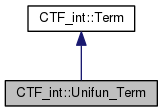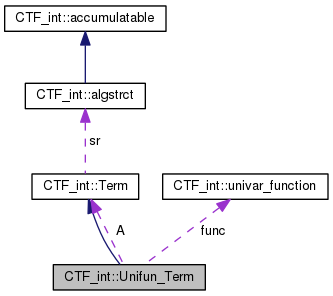Cyclops Tensor Framework parallel arithmetic on multidimensional arrays
CTF_int::Unifun_Term Class Reference

`#include <fun_term.h>`

Inheritance diagram for CTF_int::Unifun_Term:[legend]
Collaboration diagram for CTF_int::Unifun_Term:[legend]

## Public Member Functions

Unifun_Term (Term *A, univar_function const *func)

Unifun_Term (Unifun_Term const &other, std::map< tensor *, tensor * > *remap=NULL)

~Unifun_Term ()

Termclone (std::map< tensor *, tensor * > *remap=NULL) const
base classes must implement this copy function to retrieve pointer More...

void execute (CTF::Idx_Tensor output) const
evalues the expression, which just scales by default More...

CTF::Idx_Tensor execute (std::vector< char > out_inds) const
evalues the expression to produce an intermediate with all expression indices remaining More...

CTF::Idx_Tensor estimate_time (double &cost, std::vector< char > out_inds) const
estimates the cost the expression to produce an intermediate with all expression indices remaining More...

double estimate_time (CTF::Idx_Tensor output) const
estimates the cost of a contraction/sum/.. term More...

std::vector< char > get_uniq_inds () const
find list of unique indices that are involved in this term More...

void get_inputs (std::set< CTF::Idx_Tensor *, tensor_name_less > *inputs_set) const
appends the tensors this depends on to the input set More...

CTF::Worldwhere_am_i () const
figures out what world this term lives on More...Public Member Functions inherited from CTF_int::Term
Term (algstrct const *sr)

virtual ~Term ()

void mult_scl (char const *mulscl)
multiply scaling factor by mulscl More...

Contract_Term operator* (Term const &A) const
constructs a new term which multiplies by tensor A More...

Contract_Term operator* (int64_t scl) const
multiples by a constant More...

Contract_Term operator* (double scl) const

Sum_Term operator+ (Term const &A) const
constructs a new term by addition of two terms More...

Sum_Term operator+ (double scl) const

Sum_Term operator+ (int64_t scl) const

Sum_Term operator- (Term const &A) const
constructs a new term by subtracting term A More...

Sum_Term operator- (double scl) const

Sum_Term operator- (int64_t scl) const

Termoperator- ()

void operator= (CTF::Idx_Tensor const &B)
A = B, compute any operations on operand B and set. More...

void operator= (Term const &B)

void operator+= (Term const &B)

void operator-= (Term const &B)

void operator*= (Term const &B)

void operator= (double scl)

void operator+= (double scl)

void operator<< (CTF_int::Term const &B)

void operator<< (double scl)

void operator-= (double scl)

void operator*= (double scl)

void operator= (int64_t scl)

void operator+= (int64_t scl)

void operator-= (int64_t scl)

void operator*= (int64_t scl)

void operator= (int scl)

void operator+= (int scl)

void operator-= (int scl)

void operator*= (int scl)

operator float () const
cast to float (works only if tensor type is castable to float) allows a scalar output More...

operator double () const
cast to double (works only if tensor type is castable to double) allows a scalar output More...

operator int64_t () const
cast to int64_t (works only if tensor type is castable to int64_t) allows a scalar output More...

operator int () const
cast to int64_t (works only if tensor type is castable to int64_t) allows a scalar output More...

## Data Fields

TermA

univar_function const * funcData Fields inherited from CTF_int::Term
char * scale

algstrctsr

## Detailed Description

Definition at line 12 of file fun_term.h.

## Constructor & Destructor Documentation

 CTF_int::Unifun_Term::Unifun_Term ( Term * A, univar_function const * func )

Definition at line 11 of file fun_term.cxx.

References A, and func.

Referenced by clone().

 CTF_int::Unifun_Term::Unifun_Term ( Unifun_Term const & other, std::map< tensor *, tensor * > * remap = `NULL` )

Definition at line 25 of file fun_term.cxx.

 CTF_int::Unifun_Term::~Unifun_Term ( )

Definition at line 17 of file fun_term.cxx.

References A.

## Member Function Documentation

 Term * CTF_int::Unifun_Term::clone ( std::map< tensor *, tensor * > * remap = `NULL` ) const
virtual

base classes must implement this copy function to retrieve pointer

Implements CTF_int::Term.

Definition at line 21 of file fun_term.cxx.

References Unifun_Term().

 CTF::Idx_Tensor CTF_int::Unifun_Term::estimate_time ( double & cost, std::vector< char > out_inds ) const
virtual

estimates the cost the expression to produce an intermediate with all expression indices remaining

Parameters
 [in,out] cost the cost of the operation [in] out_inds unique indices to not contract/sum away
Returns
output tensor to write results into and its indices

Implements CTF_int::Term.

Definition at line 45 of file fun_term.cxx.

 double CTF_int::Unifun_Term::estimate_time ( CTF::Idx_Tensor output ) const
virtual

estimates the cost of a contraction/sum/.. term

Parameters
 [in] output tensor to write results into and its indices

Implements CTF_int::Term.

Definition at line 50 of file fun_term.cxx.

 void CTF_int::Unifun_Term::execute ( CTF::Idx_Tensor output ) const
virtual

evalues the expression, which just scales by default

Parameters
 [in,out] output tensor to write results into and its indices

Implements CTF_int::Term.

Definition at line 33 of file fun_term.cxx.

Referenced by CTF_int::univar_function::operator()().

 CTF::Idx_Tensor CTF_int::Unifun_Term::execute ( std::vector< char > out_inds ) const
virtual

evalues the expression to produce an intermediate with all expression indices remaining

Parameters
 [in] out_inds unique indices to not contract/sum away

Implements CTF_int::Term.

Definition at line 39 of file fun_term.cxx.

 void CTF_int::Unifun_Term::get_inputs ( std::set< CTF::Idx_Tensor *, tensor_name_less > * inputs_set ) const
virtual

appends the tensors this depends on to the input set

Implements CTF_int::Term.

Definition at line 62 of file fun_term.cxx.

References A, and CTF_int::Term::get_inputs().

 std::vector< char > CTF_int::Unifun_Term::get_uniq_inds ( ) const
virtual

find list of unique indices that are involved in this term

Returns
out_inds unique indices to not contract/sum away

Implements CTF_int::Term.

Definition at line 58 of file fun_term.cxx.

References A, and CTF_int::Term::get_uniq_inds().

Referenced by estimate_time(), and execute().

 CTF::World * CTF_int::Unifun_Term::where_am_i ( ) const
virtual

figures out what world this term lives on

Implements CTF_int::Term.

Definition at line 66 of file fun_term.cxx.

References A, and CTF_int::Term::where_am_i().

## Field Documentation

 Term* CTF_int::Unifun_Term::A
 univar_function const* CTF_int::Unifun_Term::func

Definition at line 15 of file fun_term.h.

Referenced by estimate_time(), execute(), and Unifun_Term().

The documentation for this class was generated from the following files: